# 2nd PUC Physics Question Bank Chapter 15 Communication Systems

Students can Download 2nd PUC Physics Chapter 15 Communication Systems Questions and Answers, Notes Pdf, 2nd PUC Physics Question Bank with Answers helps you to revise the complete Karnataka State Board Syllabus and to clear all their doubts, score well in final exams.

## Karnataka 2nd PUC Physics Question Bank Chapter 15 Communication Systems

### 2nd PUC Physics Communication Systems One Marks Questions and Answers

Question 1.
Name the Indian scientist who contributed during the initial development of radio communication?

Question 2.
Name the scientist who became successful in transmitting radio waves over long distances.
G. Marconi.Question 3.
Who proposed the theory of electromagnetic waves?
James Clerk Maxwell.

Question 4.
Who showed that electromagnetic waves can be generated and transmitted?
Heinrich Hertz.

Question 5.
Who was successful in inventing and sending telegraphic messages to a distant place?
F.B. Morse. The code was named after him as Morse Code.

Question 6.
Who invented telephone ?
Alexander Graham Bell (and Antonio Meucci).

Question 7.
Who invented World Wide Web ?
Berners Lee invented World Wide Web (WWW).

Question 8.
Name the three basic elements for communication?
Transmitter, channel or medium and receiver.

Question 9.
Name the two basic modes of communication?
The two basic modes of communication are

1. point to point and

Question 10.
Distinguish between point to point and broadcast modes of communications.
In a point to point communication mode, communication is carried over a link between a single transmitter and a receiver.
e.g.: telephony.

In a broadcast mode, there are large number of receivers corresponding to a single transmitter,

Question 11.
What is a transducer?
A transducer converts one form of energy into another.Question 12.
What is an electrical transducer?
An electrical transducer converts mechanical, thermal and optical variations into electrical variations, e.g. Capacitor microphone.

Question 13.
What is an electrical signal?
Any information converted into electrical variation and suitable for transmission is called as electrical signal.

Question 14.
Mention the two types of signals.
The two types of electrical signals are

1. analog
2. digital.

Question 15.
What are analog signals? Give an example.
Analog signals are single-valued functions of time. e.g.: sound and picture signals in TV.

Question 16.
What are digital signals?
Digital signals are double-valued signals which use zero level of voltage also called as ‘zero state’ and high voltage (+5V) as ‘logic one state’.

Question 17.
What is meant by an electrical noise?
Electrical noise is the unwanted signal which disturbs the transmission, storage and processing of message signals in a communication system,

Question 18.
What is a transmitter?
Transmitter is a device which processes the incoming message and transmits it through a medium like air or space.

Question 19.
A receiver is a device which detects, demodulates and amplifies the received message.

Question 20.
What is meant by attenuation? (March 2015)
When a signal is propagating in a medium like air or cable network, it loses its electrical strength. This loss is called attenuation.Question 21.
What is meant by modulation?
A low (audio) frequency signal varies the high frequency carrier waves, which in turn carries the audio signal into a channel or medium. This process of transmitting an AF signal with the help of a radio frequency carrier wave is called modulation.

Question 22.
What is demodulation?
The process of separation of the low (audio) frequency signal from the modulated carrier wave is called demodulation.

Question 23.
What is a repeater?
A repeater is a combination of a receiver and a transmitter. (A repeater amplifies the received signal and retransmits either with the same frequency or with a change in the carrier frequency).
e.g.: communication satellite, repeater station in space.

Question 24.
What does amplification refer to?
Amplification is a process in which a low signal or attenuated signal gets strengthened in terms of its amplitude (by using suitable electronic circuits).

Question 25.
Name the device which results in the amplification of signals.
Amplifier.

Question 26.
What refers to the range of transmission?
The range of transmission refers to the distance between the place of transmission to the place of reception.

Question 27.
What is a Bandwidth?
Bandwidth represents the range of frequencies occupied by the signal as well as the range over which an electronic equipment operates.

Question 28.
Give the Bandwidth of a TV signal. (March – 2014)
The Bandwidth of TV signal suitable for transmission is 6 MHz.

Question 29.
Give the range of audible frequencies.
Audio frequencies range from 20 Hz to 20 kHz.

Question 30.
Mention the Bandwidth of optical fiber.
The bandwidth of optical fiber is > 100 GHz. (Frequency range of 1 THz to 1000 THz is used for optical communication).
Note : 1 THz (terra Hertz) refers to microwave and 1000 THz refers to ultraviolet light.Question 31.
Mention the frequency bands of,
(iii) Television
(v) Satellite communication.
(i) 540 kHz to 1600 kHz
(ii) 88 – 108 MHz
(iii) 54 MHz – 890 MHz
(iv) 896 MHz to 901 MHz (Mobile to base station) and
840 MHz to 935 MHz (Base station to mobile)
(v) 5.925 – 6.425 GHz for uplink and 3.7 to 4,2 GHz for down link.

Question 32.
What are ground waves?
The mode of propagation of waves gliding over the ground or surface of the earth is known as surface wave propagation and these waves are called ground waves.

Question 33.
What are sky waves?
For short wave radio broadcast, the signal transmitted gets reflected by the ionosphere so that the other remote ends of the globe are capable of receiving the signals. These waves are called sky waves and the mode of propagation is called sky wave propagation.

Question 34.
What is a space wave?
A wave that travels in a straight line from the transmitting antenna to the receiving antenna, is called a space wave.

Question 35.
What are space waves used for?
Space waves are used for line-of-sight (LOS) communication and satellite communication.
Note: It is not necessary for transmitting antenna to be at the same height as that of receiving antenna for LOS communication.### 2nd PUC Physics Communication Systems Two Marks Questions and Answers

Question 1.
Write the formula connecting line of sight distance (d) in terms of the height of the transmission tower (hr) and height of receiving antenna (hR).
d = dr + drQuestion 2.
Draw a neat labelled diagram showing LOS wave, ground wave, sky wave and space wave.Question 3.
How does effective power radiated depend on the wavelength?
Power radiated ∝ $$\frac{1}{\lambda^{2}}$$

Question 4.
What is amplitude modulation?
If the amplitude of the carrier wave is varied in accordance with the information signal (audio/ video), then such modulation is called amplitude modulation.

Question 5.
Draw an amplitude modulated wave with an equation.Suffer Q-Bewdo
where, y = (Ac + Am sinωmt)sinωct
y = cm(t) – (Ac + Amsinωmt) sinωct
Ac – amplitude of carrier wave as in
c(t) – Acsin ωmt and m(t) = Amsinωmt which is a modulating signal wave
and ωc = 2 πfc and ωm = 2 πfm.Question 6.
Define modulation index. What should be its value to avoid distortion?
Modulation index is defined as the ratio between the amplitude of the modulation wave and the amplitude of the carrier wave.
μ = $$\frac{A_{m}}{A_{c}}$$
Note: μ ≤ 1 to avoid distortion.

Question 7.
Draw an amplitude modulated signal with the sidebands produced.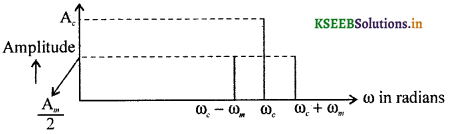Note : Sidebands are fc + fm and fc – fm and the centre frequency is fc.

Question 8.
Give the generalized block diagram of a communication system. (March 2015)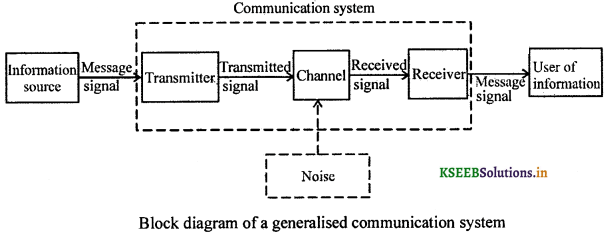Question 9.
Write the block diagram of a transmitter.Question 10.
Draw the block diagram of a receiver.Question 11.
Write the block diagram of a detector for AM signal.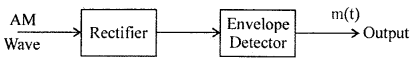Question 12.
Write the waveform at various stages of detection of the message signal. Answer: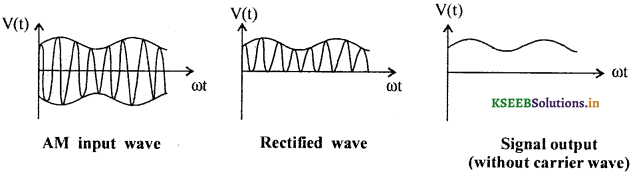Question 13.
Give the formula to calculate the range of radiation of e.m.w by an antenna.
Range dr = $$\sqrt{2 \mathrm{R} h_{\mathrm{r}}}$$ where, R = radius of the earth and hr = height of the antenna.

Question 14.
How can pulse modulation be classified?

• Pulse Amplitude Modulation (PAM)
• Pulse Duration Modulation (PDM)
• Pulse Position Modulation (PPM)
• Pulse Width Modulation (PWM)

Question 15.
What is meant by Pulse modulation?
If the information message signal modulates the pulse amplitude or pulse duration or pulse position or pulse width of the carrier wave then such modulation is called pulse modulation and the wave is called pulse modulated wave.

Question 16.
What is meant by Phase modulation?
If the message signal varies the phase of the carrier wave then such modulation is called phase modulation.

Question 17.
Mention the two types of messages that can be transmitted.
The two types of messages are

1. Analog (e.g: sinewave) and
2. Digital (e.g: Square or rectangular wave).Question 18.
Why is modulation necessary?
Low signals cannot be transmitted over long distances because of attenuation of signal, interference due to electrical noise and practical limitations of size of the transmitting and receiving antennae. Hence modulation is necessary.

Question 19.
How is Amplitude modulation produced?
Amplitude Modulation involves superposition of the message signal and the carrier wave with the help of a non linear device and application of modulated signal to a band pass filter in order to operate without interference.

Question 20.
A message signal of frequency 10 kHz and peak voltage 5V is used to modulate a carrier of frequency 100 kHz and peak voltage of 20 V. Determine
(i) Modulation index and
(ii) the side bands.
(i) Modulation index = $$\frac{A_{m}}{A_{c}}=\frac{5}{20}$$ = 0.25.
(ii) The sidebands are at (100 + 10) kHz =110 kHz and (100 – 10)kHz = 90 kHz.

Question 21.
Match the following (Inventors and their Inventions)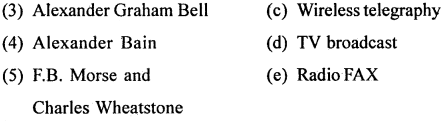1. (d)
2. (c)
3. (b)
4. (e)
5. (a)Question 22.
A carrier wave of peak voltage 12 V is used to transmit a message signal. What should be the peak voltage of the modulating signal in order to have a modulation index of 75%
MJ = 
Am = Ac(M.I)
= 12 × 0.75
= 9.00V.

Question 23.
A TV transmitting antenna is 81m tall. How much service area can it cover, if the receiving antenna is set at ground level.
Service area = πd2T
= π(2RhT)
= 3.142 × 2 × 6.4 × 106 × 81
= 3258 km2.

Question 24.
For an amplitude modulated wave, the maximum amplitude is found to be 10V while the minimum amplitude is found to be 2V. Determine the modulation index, µ.
µ = $$\frac{A_{m}}{A_{c}}$$
where, Am = Amax – Amin = 10 – 12 = 8
Ac = Amax + Amin = 10 + 2 = 12
∴ µ = $$\frac{8}{12}=\frac{2}{3}$$

### 2nd PUC Physics Communication Systems Three Marks Questions and Answers

Question 1.
Outline the production of an Amplitude Modulated Wave.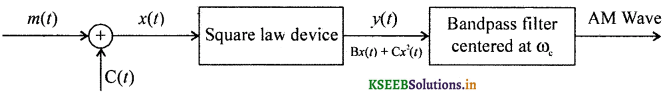Theory : Let m(t) = Amsinωmt and c(t) = Acsinωct, where m{t) is a message signal and c(t) is the carrier wave. On modulation, the resultant wave becomes x(t) = Amsinωmt + Acsinωct (Theorem of superposition of waves). The combined wave is applied to a non linear device called square law device which produces the output y(t) = Bx(t) + Cx2(t) where B and C are constants. On simplification we get,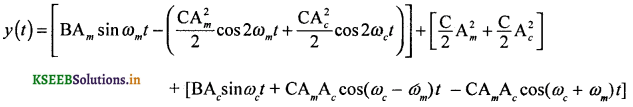If signal y(t) is passed through the band pass filter centered at ωc, it rejects the sine terms of ωm, 2ω,m and 2ωc (I term) and the DC terms (II term). The output of the band pass filter consists of only ωc, ωc + ωm and ωc – ωm. This output is the modulated carrier wave (IH term) which is further amplified before transmitting.

Question 2.
Why there is a need for modulation? (March 2014)
There is a need for modulation in order to

1. reduce the size of the antenna/aerial.
2. increase the effective power radiated by antenna.
3. avoid mixing up of signals from different transmitters.Question 3.
Outline the detection of an Amplitude Modulated Wave.
The transmitted signal loses energy travelling through a medium (attenuation). The receiver consists of an amplifier which amplifies the modulated carrier wave. The local oscillator produces a variable frequency range so that on selection of broadcast signal frequency, the receiver produces a beat frequency called intermediate frequency which remains a constant irrespective of broadcast frequencies. The detector separates the carrier frequency from the low frequency which is then sufficiently amplified before connecting to the transducer.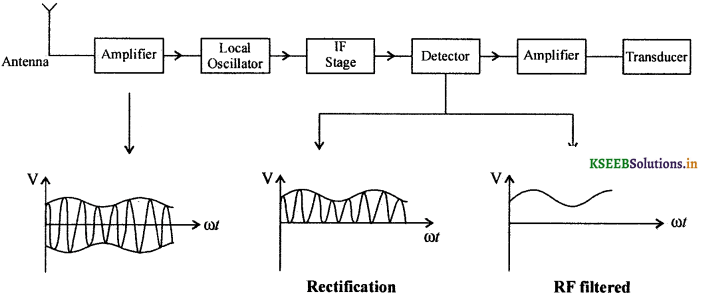Question 4.
If only the upper side band of an AM wave is transmitted and there is a facility for generating the carrier at the receiving end then is it possible to recover the modulating signal at the receiver station if a device is available for multiplying two signals? Explain.
= $$\frac{A_{1} A_{c}}{2}$$[cos(2ωc) + ωm)t + cosωmt]
m(t) = $$\frac{A_{c} A_{c}}{2}$$cos ωmt